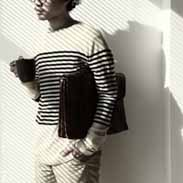# Biology Topic 2 Lab Tools and Lab SkillsChad Lipe
question

centrifuge

separates mixtures based on density
question

microscope

helps scientists see things they can’t with the naked eye
question

simple light microscope

uses 1 lens to form an image and 1 eyepiece
question

compound light microscope

uses 2 lenses to form an image and 1 eyepiece
question

simple and compound light microscopes

image is reversed
question

stereo dissecting microscope

produces 3D images of specimens image is not reversed and 2 eyepieces
question

electron microscope

shines a beam of electrons through the specimen that must be dead before being viewed
question

metric system

the system of measurement used by scientists worldwide
question

10

the number that the multiples of the metric system are based on
question

length

how long something is
question

mass

the amount of matter in an object
question

volume

the amount of space something takes up
question

temperature

the amount of heat in an object
question

metric ruler

used to measure length
question

balance

used to measure mass
question

used to measure the volume of liquids
question

thermometer

used to measure temperature
question

meter

the metric unit for length
question

gram

the metric unit for mass
question

liter

the metric unit for volume
question

celsius

the metric unit for temperature
question

meniscus

the concave surface of a liquid in a graduated cylinder
question

mm

millimeters (are the lines that are one tenth each number on the metric ruler)
question

cm

centimeters (are the lines that are each number on the metric ruler)
question

eyepiece

contains the ocular lens
question

revolving nosepiece

holds the objective lenses and can be rotated to change magnification
question

objective lenses

magnification ranges from 4 times to 40 times
question

stage clips

hold the slide in place
question

stage

supports the slide being viewed
question

light source

projects light upwards through the diaphragm the specimen and the lenses
question

arm

used to support the microscope when carried
question

moves the stage up and down for focusing
question

moves the stage slightly to sharpen the image
question

diaphragm

regulates the amount of light on the specimen
question

base

supports the microscope
question

total magnification

eyepiece magnification times objective magnification
question

microscope field of view

the area you see when you look into a microscope
question

staining

a technique used to make cell parts more visible
question

pH

the scale used to determine if a solution is an acid a base or a neutral
question

litmus paper

determines whether solutions are an acid or a base
question

how litmus paper works

an acid turns blue litmus paper red and a base turns red litmus paper blue a neutral does not change the color
question

pH paper

is used to determine the actual pH of a solution
question

how pH paper works

the paper is dipped in the solution and it turns a color that is then compared to a chart that shows the pH
question

color and the pH scale

the pH scale is 0 to 14 where 7 is neutral 0 to 6 is an acid and 8 to 14 is a base, the colors are neutral is greenish-yellow an acid is red to yellow and a base is green to violet
question

Bromthymol blue

turns yellow in the presence of carbon dioxide
question

Benedict’s solution

turns from blue to yellow orange brick red in the presence of glucose
question

Lugol’s iodine

turns from amber to blue-black in the presence of starch used for the staining of plant cells
question

graphs

help us visualize numerical data in an organized way that is easeier to interpret
question

bar graphs

used to show a comparison between multiple objects
question

pie graphs

used to compare parts of a whole
question

line graphs

used to show the relationship between variables
question

direct relationship

as x increases y increases
question

inverse relationship

as x increases y decreases
question

constant relationship

as x increases y remains the same
question

independent variable

is always on the x-axis
question

dependent variable

is always on the y-axis
question

phase contrast microscope

enhances and contrasts the image of transparent objects
question

Phenolphthalein solution

turns from clear to bright pink in the presence of either carbon dioxide or a base
question

Biuret solution